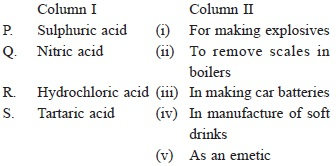# User Forum

Subject :NSO    Class : Class 7

Match column I with column II and select the correct option form the given codes.AP-(ii), Q-(iii), R-(v), S-(iv)
BP-(iii), Q-(v), R-(ii), S-(iv)
CP-(iii), Q-(i), R-(ii), S-(v)
DP-(ii), Q-(iii), R-(i), S-(v)